GATE  >  Practice Test: Gate Civil Engineering(CE) 2015 Paper

# Practice Test: Gate Civil Engineering(CE) 2015 Paper

Test Description

## 65 Questions MCQ Test GATE Past Year Papers for Practice (All Branches) | Practice Test: Gate Civil Engineering(CE) 2015 Paper

Practice Test: Gate Civil Engineering(CE) 2015 Paper for GATE 2023 is part of GATE Past Year Papers for Practice (All Branches) preparation. The Practice Test: Gate Civil Engineering(CE) 2015 Paper questions and answers have been prepared according to the GATE exam syllabus.The Practice Test: Gate Civil Engineering(CE) 2015 Paper MCQs are made for GATE 2023 Exam. Find important definitions, questions, notes, meanings, examples, exercises, MCQs and online tests for Practice Test: Gate Civil Engineering(CE) 2015 Paper below.
Solutions of Practice Test: Gate Civil Engineering(CE) 2015 Paper questions in English are available as part of our GATE Past Year Papers for Practice (All Branches) for GATE & Practice Test: Gate Civil Engineering(CE) 2015 Paper solutions in Hindi for GATE Past Year Papers for Practice (All Branches) course. Download more important topics, notes, lectures and mock test series for GATE Exam by signing up for free. Attempt Practice Test: Gate Civil Engineering(CE) 2015 Paper | 65 questions in 180 minutes | Mock test for GATE preparation | Free important questions MCQ to study GATE Past Year Papers for Practice (All Branches) for GATE Exam | Download free PDF with solutions
 1 Crore+ students have signed up on EduRev. Have you?
Practice Test: Gate Civil Engineering(CE) 2015 Paper - Question 1

### Q. 1 – Q. 5 carry one mark each.  Extreme focus on syllabus and studying for test has become sach a dominant concern of Indian students that they close their mind to anything __________________ to the requirments of the exam.

Practice Test: Gate Civil Engineering(CE) 2015 Paper - Question 2

### Select the pair that best express es a relationship similar to that expressed in the pair : Childern : Pediatrician

Detailed Solution for Practice Test: Gate Civil Engineering(CE) 2015 Paper - Question 2

Pediatricians are doctors who manage the health of your child, including physical, behavior, and mental health issues.
An orthopaedic surgeon, a doctor who corrects congenital or functional abnormalities of the bones with surgery, casting, and bracing.
Gynecologists are doctors who specialize in women's health, with a focus on the female reproductive system
A nephrologist is a type of doctor that specializes in diseases and conditions that affect the kidneys.
A dermatologist is a medical practitioner qualified to diagnose and treat skin disorders
Here, in the question we are comparing the type of human and branch of medical science which specialises in dealing with it and not with the type of organ and branch of medical science that specialises in it.

Practice Test: Gate Civil Engineering(CE) 2015 Paper - Question 3

### The Tamil version of ___________ John Abraham-strrer Madras Cafe____________cleared by the Censor Board with no cuts last week, but the film's distributors_____________no takers amogn the exhibitors for a release in Tamil Nadu_____________this Friday.

Practice Test: Gate Civil Engineering(CE) 2015 Paper - Question 4

If ROAD is written as URDG, the SWAN should be written as:

Detailed Solution for Practice Test: Gate Civil Engineering(CE) 2015 Paper - Question 4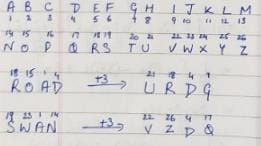Practice Test: Gate Civil Engineering(CE) 2015 Paper - Question 5

A function f(x) is linear and has a value of 29 at x= -2 and 39 at x=3. Find its value at x=5.

Detailed Solution for Practice Test: Gate Civil Engineering(CE) 2015 Paper - Question 5Practice Test: Gate Civil Engineering(CE) 2015 Paper - Question 6

Q. 6 – Q. 10 carry Two mark each.

Alexender turned his attetion towrds India, since he had conquered Persia.

Which one of the statements below is logically valid and can be inferred from the above sentence?

Detailed Solution for Practice Test: Gate Civil Engineering(CE) 2015 Paper - Question 6

The statement represented is of type action because of a reason. Had reason was not been able to complete action would not have taken place.
Thus, the conclusion from the statement can be Alexander would not have turned his attention towards India had he not conquered India.

Practice Test: Gate Civil Engineering(CE) 2015 Paper - Question 7

Most experts feel that in spite of possessing all the technical skills required to be a batsman of the highest order, he is unlikely to be so due to lack of requisite temperament. he was guilty of throwing away his wicket several times after working hard to lay a strong foundation. His critics pointed out that until he addressed this problem, success at the highest level will contiunue to elude him.

Which of the statements(s) below is/are logically valid and can be inferred from the above passage?

i. He was already a successful batsman at the highest level.

ii. He has to improve his temperament in order to became a great batsman.

iii. he failed to make many of his good starts count.

iv. Improving his technical skills will gurantee success.

*Answer can only contain numeric values
Practice Test: Gate Civil Engineering(CE) 2015 Paper - Question 8

The exporst and imports (in crores of Rs.) of a country from the year 2000 to 2007 are given in the following bar chart. In which year is the combined percentage icncrease in impoerts and exports the highest?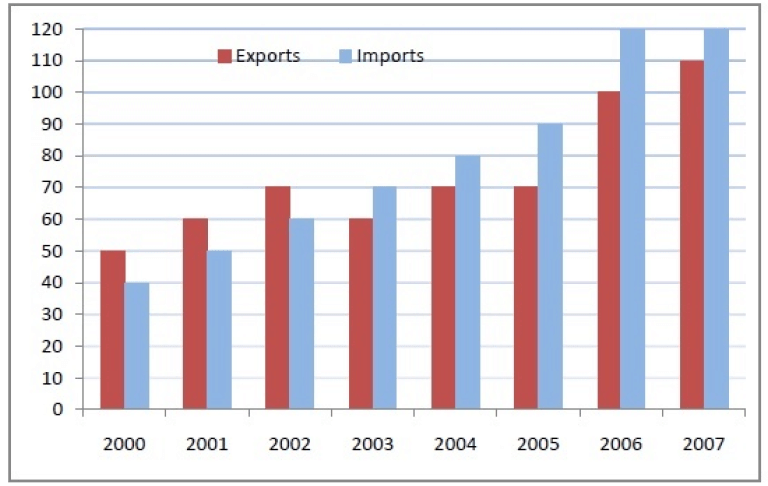Detailed Solution for Practice Test: Gate Civil Engineering(CE) 2015 Paper - Question 8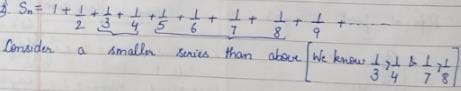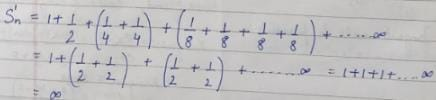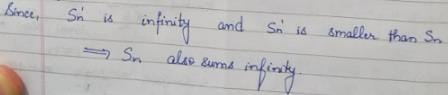Practice Test: Gate Civil Engineering(CE) 2015 Paper - Question 9

Choose the most appropriate equation for the function drwan as a thick line, in the plot below.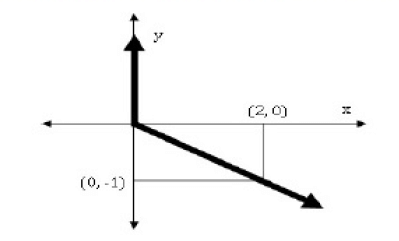Detailed Solution for Practice Test: Gate Civil Engineering(CE) 2015 Paper - Question 9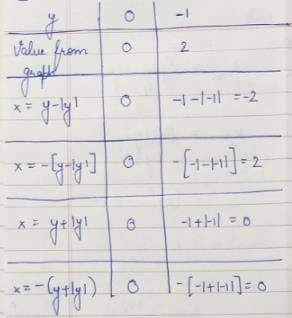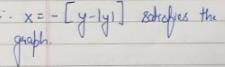Practice Test: Gate Civil Engineering(CE) 2015 Paper - Question 10

The head of anewly formed government desires to appoint five of the six selected members P,Q,R,S,T and U to portfolios of Home, Power, Defense, Telecom, and Finance. U does not want any portfolio if S gets one of the feive. R wants either Home or Finance or no portfolio. Q says that if S gets either Power or Telecom, then she must get the other one. T insists on a portfolio if P gets one.

Which is the valid distribution of portfolios?

*Answer can only contain numeric values
Practice Test: Gate Civil Engineering(CE) 2015 Paper - Question 11

Q. 11 – Q. 35 carry one mark each.

For what value of p the following set of equations will have no solution?
2x + 3y = 5
3x + py = 10

Detailed Solution for Practice Test: Gate Civil Engineering(CE) 2015 Paper - Question 11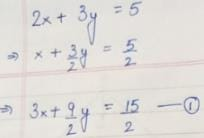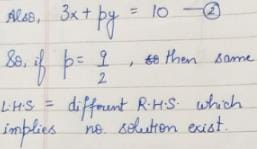Practice Test: Gate Civil Engineering(CE) 2015 Paper - Question 12

The integral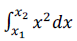with x2 >x1 > 0 is evaluated analytically as well as numerically using a single application of the trapezoidal rule. If I is the exact value of the integral obtained analytically and J is the approximate value obtained using the trapezoidal rule, which of the following statements is correct about their relationship?

Detailed Solution for Practice Test: Gate Civil Engineering(CE) 2015 Paper - Question 12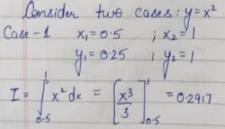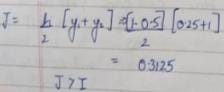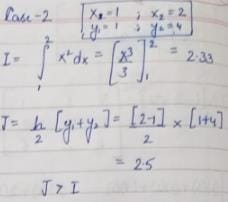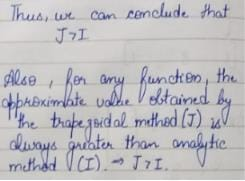*Answer can only contain numeric values
Practice Test: Gate Civil Engineering(CE) 2015 Paper - Question 13

Consider the following probability mass function (p.m.f.) of a random variable X: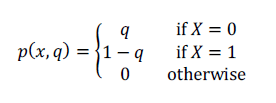If q = 0.4, the variance of X is _______________.

Detailed Solution for Practice Test: Gate Civil Engineering(CE) 2015 Paper - Question 13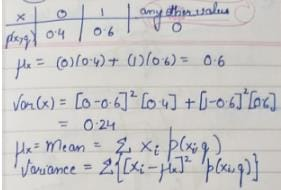Practice Test: Gate Civil Engineering(CE) 2015 Paper - Question 14

Workability of concrete can be measured using slump, compaction factor and Vebe time. Consider the following statements for workability of concrete:

(i) As the slump increases, the Vebe time increases
(ii) As the slump increases, the compaction factor increases

Which of the following is TRUE?

Practice Test: Gate Civil Engineering(CE) 2015 Paper - Question 15

Consider the following statements for air-entrained concrete:

(i) Air-entrainment reduces the water demand for a given level of workability
(ii) Use of air-entrained concrete is required in environments where cyclic freezing and thawing is expected

Which of the following is TRUE?

Practice Test: Gate Civil Engineering(CE) 2015 Paper - Question 16

Consider the singly reinforced beam shown in the figure below: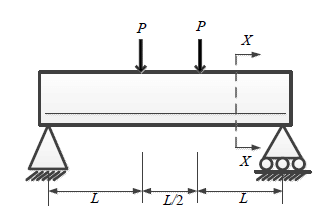At cross-section XX, which of the following statements is TRUE at the limit state?

Detailed Solution for Practice Test: Gate Civil Engineering(CE) 2015 Paper - Question 16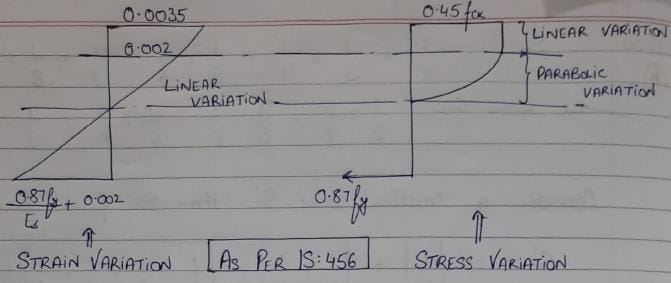Practice Test: Gate Civil Engineering(CE) 2015 Paper - Question 17

For the beam shown below, the stiffness coefficient K22 can be written as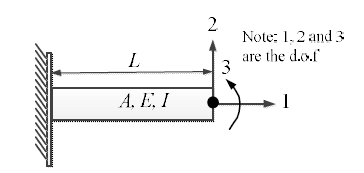Detailed Solution for Practice Test: Gate Civil Engineering(CE) 2015 Paper - Question 17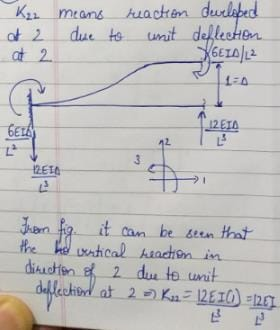*Answer can only contain numeric values
Practice Test: Gate Civil Engineering(CE) 2015 Paper - Question 18

The development length of a deformed reinforcement bar can be expressed as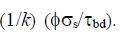From the IS:456-2000, the value of K can be calculated as _______________.

*Answer can only contain numeric values
Practice Test: Gate Civil Engineering(CE) 2015 Paper - Question 19

For the beam shown below, the value of the support moment M is _______________ kN-m.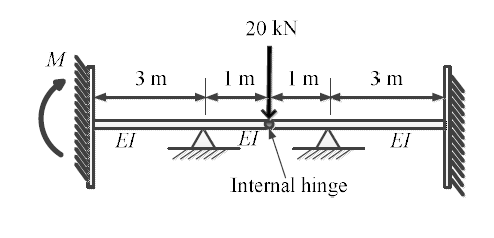Detailed Solution for Practice Test: Gate Civil Engineering(CE) 2015 Paper - Question 19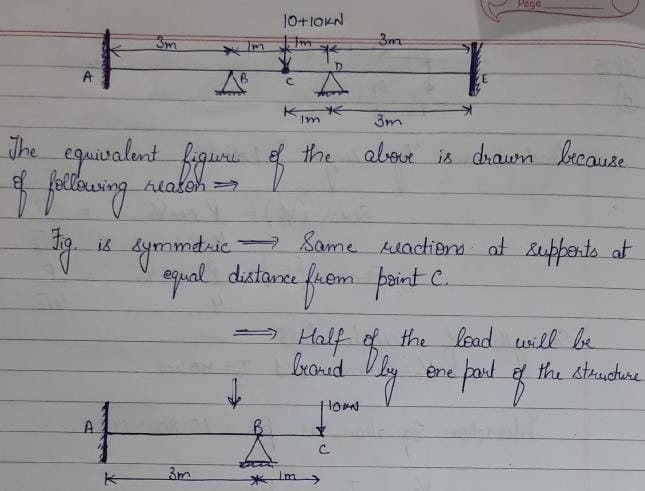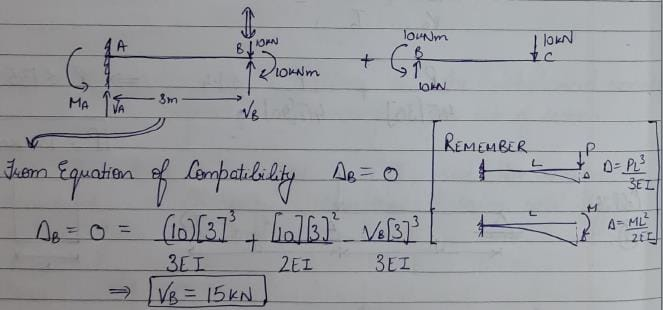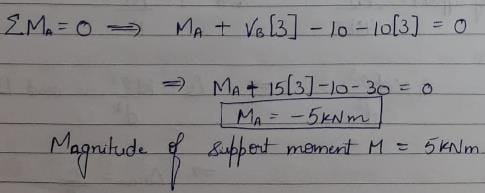*Answer can only contain numeric values
Practice Test: Gate Civil Engineering(CE) 2015 Paper - Question 20

Two triangular wedges are glued together as shown in the following figure. The stress acting normal to the interface, σn is _______________ MPa.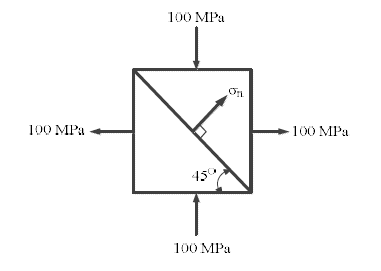Practice Test: Gate Civil Engineering(CE) 2015 Paper - Question 21

A fine grained soil has 60%(by weight) silt content. The soil behaves as semi-solid when water content is between 15% and 28%. The soil behaves fluid-like when the water content is more than 40%. The activity of the soil is

Practice Test: Gate Civil Engineering(CE) 2015 Paper - Question 22

Which of the following statements is TRUE for the relation between discharge velocity and seepage velocity?

Practice Test: Gate Civil Engineering(CE) 2015 Paper - Question 23

Which of the following statements is TRUE for degree of disturbance of collected soil sample?

Practice Test: Gate Civil Engineering(CE) 2015 Paper - Question 24

In an unconsolidated undrained triaxial test, it is observed that an increase in cell pressure from 150 kPa to 250 kPa leads to a pore pressure increase of 80 kPa. It is further observed that, an increase of 50 kPa in deviatoric stress results in an increase of 25 kPa in the pore pressure. The value of Skempton’s pore pressure parameter B is:

Practice Test: Gate Civil Engineering(CE) 2015 Paper - Question 25

Which of the following statements is NOT correct?

Practice Test: Gate Civil Engineering(CE) 2015 Paper - Question 26

In a two-dimensional steady flow field, in a certain region of the x-y plane, the velocity component in the x-direction is given by vx = xand the density varies as ρ = 1/x . Which of the following is a valid expression for the velocity component in the y-direction, vy?

Detailed Solution for Practice Test: Gate Civil Engineering(CE) 2015 Paper - Question 26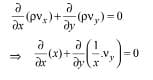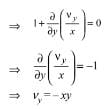Practice Test: Gate Civil Engineering(CE) 2015 Paper - Question 27

For steady incompressible flow through a closed-conduit of uniform cross-section, the direction of flow will always be:

Practice Test: Gate Civil Engineering(CE) 2015 Paper - Question 28

A circular pipe has a diameter of 1 m, bed slope of 1 in 1000, and Manning’s roughness coefficient equal to 0.01. It may be treated as an open channel flow when it is flowing just full, i.e., the water level just touches the crest. The discharge in this condition is denoted by Qfull. Similarly, the discharge when the pipe is flowing half-full, i.e., with a flow depth of 0.5 m, is denoted by Qhalf. The ratio Qfull / Qhalf is:

Practice Test: Gate Civil Engineering(CE) 2015 Paper - Question 29

The two columns below show some parameters and their possible values.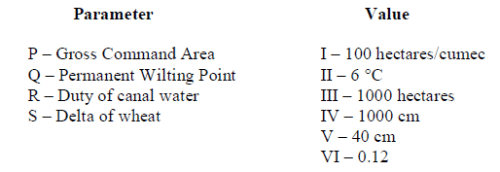Which of the following options matches the parameters and the values correctly?

Practice Test: Gate Civil Engineering(CE) 2015 Paper - Question 30

Total Kjeldahl Nitrogen (TKN) concentration (mg/L as N) in domestic sewage is the sum of the concentrations of:

Practice Test: Gate Civil Engineering(CE) 2015 Paper - Question 31

Solid waste generated from an industry contains only two components, X and Y as shown in the
table below
Component                        Composition                    Density
(% weight)                     (kg/m3)
X                                          c1                               ρ1
Y                                          c2                               ρ2

Assuming (c1 + c2) = 100, the composite density of the solid waste ( ρ is given by:

Detailed Solution for Practice Test: Gate Civil Engineering(CE) 2015 Paper - Question 31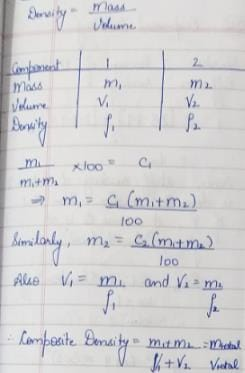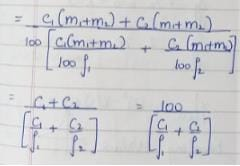*Answer can only contain numeric values
Practice Test: Gate Civil Engineering(CE) 2015 Paper - Question 32

The penetration value of a bitumen sample tested at 25°C is 80. When this sample is heated to 60°C and tested again, the needle of the penetration test apparatus penetrates the bitumen sample by d mm. The value of d CANNOT be less than _______________ mm.

Practice Test: Gate Civil Engineering(CE) 2015 Paper - Question 33

Which of the following statements CANNOT be used to describe free flow speed (uf) of a traffic stream?

Practice Test: Gate Civil Engineering(CE) 2015 Paper - Question 34

Which of the following statements is FALSE?

Practice Test: Gate Civil Engineering(CE) 2015 Paper - Question 35

In a closed loop traverse of 1 km total length, the closing errors in departure and latitude are 0.3 m and 0.4 m, respectively. The relative precision of this traverse will be:

Practice Test: Gate Civil Engineering(CE) 2015 Paper - Question 36

Q. 36 – Q. 65 carry two marks each.

The smallest and largest Eigen values of the following matrix are: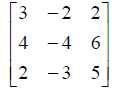Detailed Solution for Practice Test: Gate Civil Engineering(CE) 2015 Paper - Question 36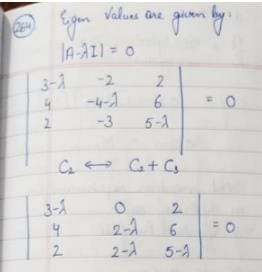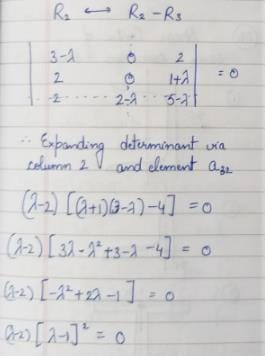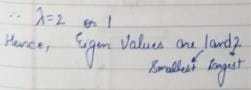*Answer can only contain numeric values
Practice Test: Gate Civil Engineering(CE) 2015 Paper - Question 37

The quadratic equation x2 − 4x + 4 = 0 is to be solved numerically, starting with the initial guess xo = 3. The Newton-Raphson method is applied once to get a new estimate and then the Secant method is applied once using the initial guess and this new estimate. The estimated value of the root after the application of the Secant method is _______________.

Practice Test: Gate Civil Engineering(CE) 2015 Paper - Question 38

Consider the following differential equation: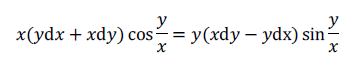Which of the following is the solution of the above equation (c is an arbitrary constant)?

Practice Test: Gate Civil Engineering(CE) 2015 Paper - Question 39

Consider the following complex function: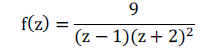Which of the following is one of the residues of the above function?

*Answer can only contain numeric values
Practice Test: Gate Civil Engineering(CE) 2015 Paper - Question 40

The directional derivative of the field u(x, y, z) = x2 − 3yz in the direction of the vector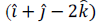at point (2, −1, 4) is _______________.

Detailed Solution for Practice Test: Gate Civil Engineering(CE) 2015 Paper - Question 40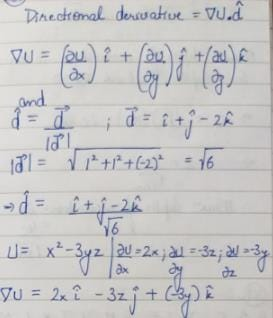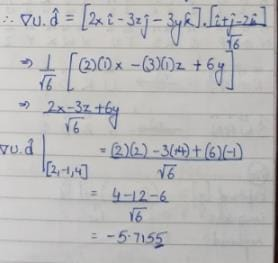*Answer can only contain numeric values
Practice Test: Gate Civil Engineering(CE) 2015 Paper - Question 41

A bracket plate connected to a column flange transmits a load of 100 kN as shown in the following figure. The maximum force for which the bolts should be designed is _______________ kN.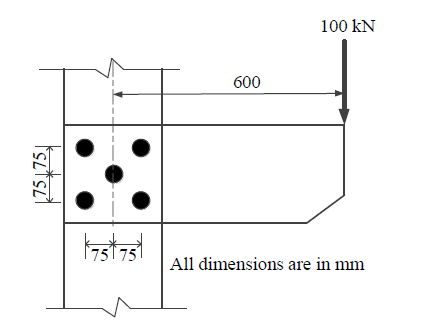*Answer can only contain numeric values
Practice Test: Gate Civil Engineering(CE) 2015 Paper - Question 42

The composition of an air-entrained concrete is given below: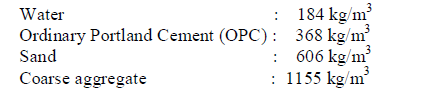Assume the specific gravity of OPC, sand and coarse aggregate to be 3.14, 2.67 and 2.74, respectively. The air content is _______________ liters/ m3

*Answer can only contain numeric values
Practice Test: Gate Civil Engineering(CE) 2015 Paper - Question 43

Consider the singly reinforced beam section given below (left figure). The stress block parameters for the cross-section from IS:456-2000 are also given below (right figure). The moment of resistance for the given section by the limit state method is _______________ kN-m.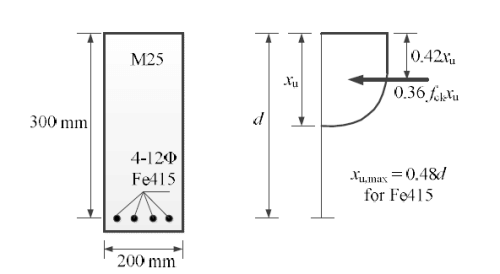*Answer can only contain numeric values
Practice Test: Gate Civil Engineering(CE) 2015 Paper - Question 44

For formation of collapse mechanism in the following figure, the minimum value of Pu is cMp/L. Mp and 3Mp denote the plastic moment capacities of beam sections as shown in this figure. The value of c is _______________.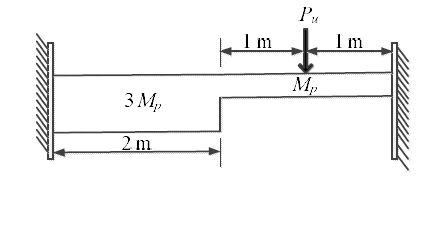*Answer can only contain numeric values
Practice Test: Gate Civil Engineering(CE) 2015 Paper - Question 45

A tapered circular rod of diameter varying from 20 mm to 10 mm is connected to another uniform circular rod of diameter 10 mm as shown in the following figure. Both bars are made of same material with the modulus of elasticity, E = 2x105 MPa. When subjected to a load P = 30π kN, the deflection at point A is _______________ mm.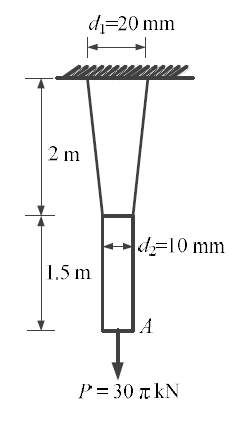*Answer can only contain numeric values
Practice Test: Gate Civil Engineering(CE) 2015 Paper - Question 46

Two beams are connected by a linear spring as shown in the following figure. For a load P as shown in the figure, the percentage of the applied load P carried by the spring is _______________.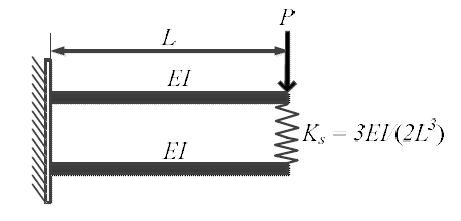Detailed Solution for Practice Test: Gate Civil Engineering(CE) 2015 Paper - Question 46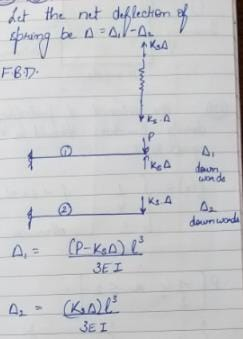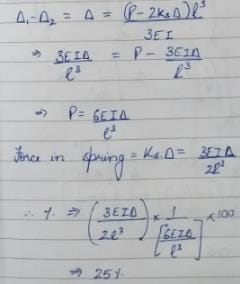*Answer can only contain numeric values
Practice Test: Gate Civil Engineering(CE) 2015 Paper - Question 47

For the 2D truss with the applied loads shown below, the strain energy in the member XY is _______________ kN-m. For member XY, assume AE = 30 kN, where A is cross-section area and E is the modulus of elasticity.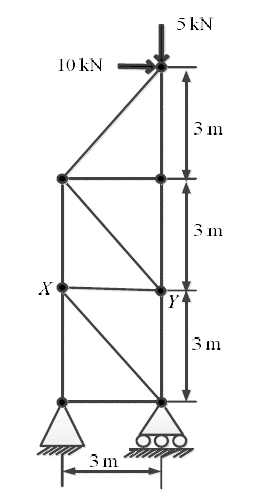Practice Test: Gate Civil Engineering(CE) 2015 Paper - Question 48

An earth embankment is to be constructed with compacted cohesionless soil. The volume of the embankment is 5000 m3 and the target dry unit weight is 16.2 kN/m3. Three nearby sites (see figure below) have been identified from where the required soil can be transported to the construction site. The void ratios (e) of different sites are shown in the figure. Assume the specific gravity of soil to be 2.7 for all three sites. If the cost of transportation per km is twice the cost of excavation per m3 of borrow pits, which site would you choose as the most economic solution? (Use unit weight of water = 10 kN/m3)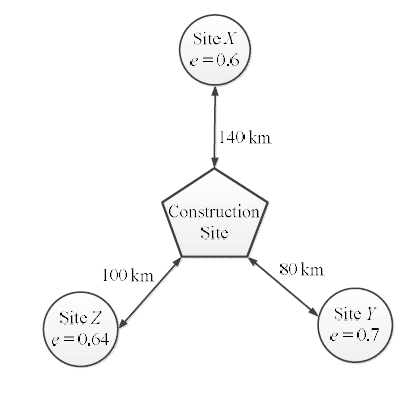*Answer can only contain numeric values
Practice Test: Gate Civil Engineering(CE) 2015 Paper - Question 49

A water tank is to be constructed on the soil deposit shown in the figure below. A circular footing of diameter 3 m and depth of embedment 1 m has been designed to support the tank. The total vertical load to be taken by the footing is 1500 kN. Assume the unit weight of water as 10 kN/m3 and the load dispersion pattern as 2V:1H. The expected settlement of the tank due to primary consolidation of the clay layer is _______________ mm.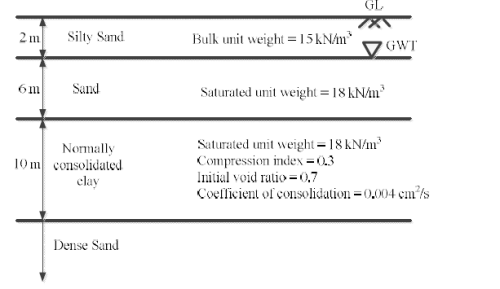*Answer can only contain numeric values
Practice Test: Gate Civil Engineering(CE) 2015 Paper - Question 50

A 20 m thick clay layer is sandwiched between a silty sand layer and a gravelly sand layer. The layer experiences 30 mm settlement in 2 years.

Given: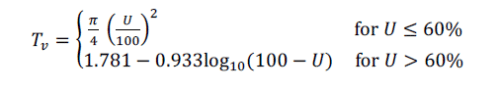where Tv is the time factor and U is the degree of consolidation in %.

If the coefficient of consolidation of the layer is 0.003 cm2/s, the deposit will experience a total of 50 mm settlement in the next _______________ years.

*Answer can only contain numeric values
Practice Test: Gate Civil Engineering(CE) 2015 Paper - Question 51

A non-homogeneous soil deposit consists of a silt layer sandwiched between a fine-sand layer at top and a clay layer below. Permeability of the silt layer is 10 times the permeability of the clay layer and one-tenth of the permeability of the sand layer. Thickness of the silt layer is 2 times the thickness of the sand layer and two-third of the thickness of the clay layer. The ratio of equivalent horizontal and equivalent vertical permeability of the deposit is ___________________.

Detailed Solution for Practice Test: Gate Civil Engineering(CE) 2015 Paper - Question 51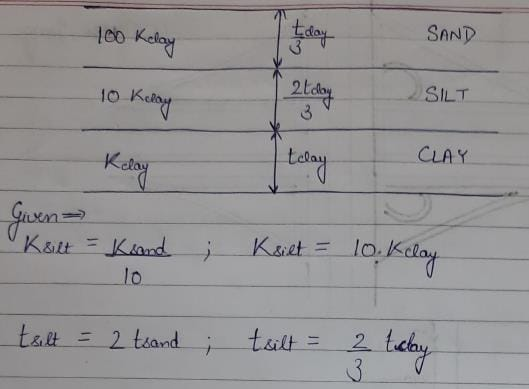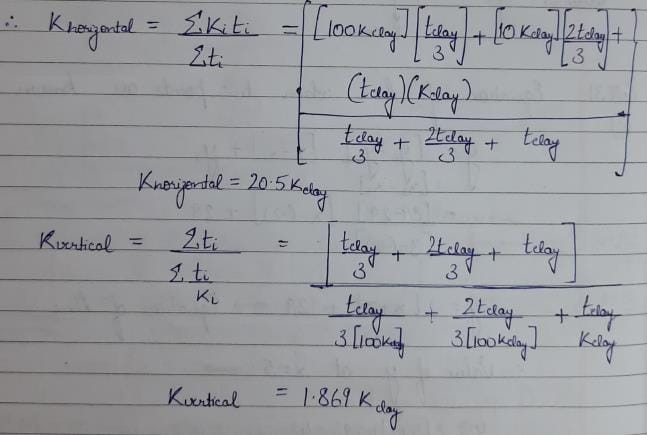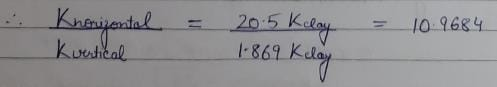*Answer can only contain numeric values
Practice Test: Gate Civil Engineering(CE) 2015 Paper - Question 52

A square footing (2 m x 2 m) is subjected to an inclined point load, P as shown in the figure below. The water table is located well below the base of the footing. Considering one-way eccentricity, the net safe load carrying capacity of the footing for a factor of safety of 3.0 is _______________ kN.

The following factors may be used:

Bearing capacity factors: Nq = 33.3, Nγ= 37.16 Shape factors: Fqs = Fγs = 1.314; Depth factors: Fqd = Fγd = 1.113; Inclination factors: Fqi = 0.444, Fγi = 0.02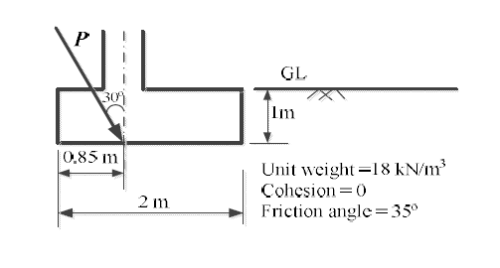*Answer can only contain numeric values
Practice Test: Gate Civil Engineering(CE) 2015 Paper - Question 53

Two reservoirs are connected through a 930 m long, 0.3 m diameter pipe, which has a gate valve. The pipe entrance is sharp (loss coefficient = 0.5) and the valve is half-open (loss coefficient = 5.5). The head difference between the two reservoirs is 20 m. Assume the friction factor for the pipe as 0.03 and g =10 m/s2. The discharge in the pipe accounting for all minor and major losses is _______________ m3/s.

*Answer can only contain numeric values
Practice Test: Gate Civil Engineering(CE) 2015 Paper - Question 54

A hydraulic jump is formed in a 2 m wide rectangular channel which is horizontal and frictionless. The post-jump depth and velocity are 0.8 m and 1 m/s, respectively. The pre-jump velocity is _______________ m/s. (use g = 10 m/s2)

*Answer can only contain numeric values
Practice Test: Gate Civil Engineering(CE) 2015 Paper - Question 55

A short reach of a 2 m wide rectangular open channel has its bed level rising in the direction of flow at a slope of 1 in 10000. It carries a discharge of 4 m3/s and its Manning’s roughness coefficient is 0.01. The flow in this reach is gradually varying. At a certain section in this reach, the depth of flow was measured as 0.5 m. The rate of change of the water depth with distance, dy/dx, at this section is _______________ (use g = 10 m/s2).

Practice Test: Gate Civil Engineering(CE) 2015 Paper - Question 56

The drag force, FD, on a sphere kept in a uniform flow field depends on the diameter of the sphere, D; flow velocity, V; fluid density, ρ; and dynamic viscosity, μ. Which of the following options represents the non-dimensional parameters which could be used to analyze this problem?

*Answer can only contain numeric values
Practice Test: Gate Civil Engineering(CE) 2015 Paper - Question 57

In a catchment, there are four rain-gauge stations, P, Q, R, and S. Normal annual precipitation values at these stations are 780 mm, 850 mm, 920 mm, and 980 mm, respectively. In the year 2013, stations Q, R, and S, were operative but P was not. Using the normal ratio method, the precipitation at station P for the year 2013 has been estimated as 860 mm. If the observed precipitation at stations Q and R for the year 2013 were 930 mm and 1010 mm, respectively; what was the observed precipitation (in mm) at station S for that year?

*Answer can only contain numeric values
Practice Test: Gate Civil Engineering(CE) 2015 Paper - Question 58

The 4-hr unit hydrograph for a catchment is given in the table below. What would be the maximum ordinate of the S-curve (in m3/s) derived from this hydrograph?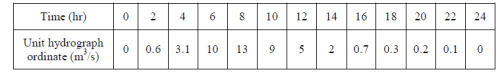*Answer can only contain numeric values
Practice Test: Gate Civil Engineering(CE) 2015 Paper - Question 59

The concentration of Sulfur Dioxide (SO2) in ambient atmosphere was measured as 30 μg/m3. Under the same conditions, the above SO2 concentration expressed in ppm is _______________.

Given: P/(RT) = 41.6mol/m3; where, P = Pressure; T = Temperature; R = universal gas constant; Molecular weight of SO2 = 64.

*Answer can only contain numeric values
Practice Test: Gate Civil Engineering(CE) 2015 Paper - Question 60

Consider a primary sedimentation tank (PST) in a water treatment plant with Surface Overflow Rate (SOR) of 40 m3/m2/d. The diameter of the spherical particle which will have 90 percent theoretical removal efficiency in this tank is _______________ μm. Assume that settling velocity of the particles in water is described by Stokes’s Law.

Given: Density of water = 1000 kg/m3; Density of particle = 2650 kg/m3; g = 9.81 m/s2; Kinematic viscosity of water (v)= 1.10 x 10-6 m2/s

*Answer can only contain numeric values
Practice Test: Gate Civil Engineering(CE) 2015 Paper - Question 61

The acceleration-time relationship for a vehicle subjected to non-uniform acceleration is,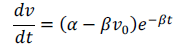where, v is the speed in m/s, t is the time in s, α and β are parameters, and v0 is the initial speed in m/s. If the accelerating behavior of a vehicle, whose driver intends to overtake a slow moving vehicle ahead, is described as,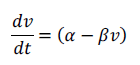Considering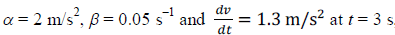, distance (in m) travelled by
the vehicle in 35 s is ______________.

Practice Test: Gate Civil Engineering(CE) 2015 Paper - Question 62

On a circular curve, the rate of superelevation is e. While negotiating the curve a vehicle comes to a stop. It is seen that the stopped vehicle does not slide inwards (in the radial direction). The coefficient of side friction is f. Which of the following is true:

Detailed Solution for Practice Test: Gate Civil Engineering(CE) 2015 Paper - Question 62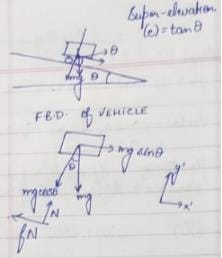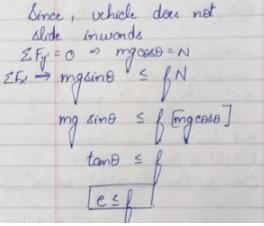*Answer can only contain numeric values
Practice Test: Gate Civil Engineering(CE) 2015 Paper - Question 63

A sign is required to be put up asking drivers to slow down to 30 km/h before entering Zone Y (see figure). On this road, vehicles require 174 m to slow down to 30 km/h (the distance of 174 m includes the distance travelled during the perception-reaction time of drivers). The sign can be read by 6/6 vision drivers from a distance of 48 m. The sign is placed at a distance of x m from the start of Zone Y so that even a 6/9 vision driver can slow down to 30 km/h before entering the zone. The minimum value of x is _______________ m.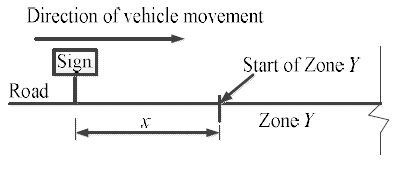Practice Test: Gate Civil Engineering(CE) 2015 Paper - Question 64

In a survey work, three independent angles X, Y and Z were observed with weights WX, WY, WZ, respectively. The weight of the sum of angles X, Y and Z is given by:

Practice Test: Gate Civil Engineering(CE) 2015 Paper - Question 65

In a region with magnetic declination of 2oE, the magnetic Fore bearing (FB) of a line AB was measured as N79o50' E. There was local attraction at A. To determine the correct magnetic bearing of the line, a point O was selected at which there was no local attraction. The magnetic FB of line AO and OA were observed to be S52o40'E and N50o20'W, respectively. What is the true FB of line AB?

## GATE Past Year Papers for Practice (All Branches)

407 docs|127 tests
 Use Code STAYHOME200 and get INR 200 additional OFF Use Coupon Code
Information about Practice Test: Gate Civil Engineering(CE) 2015 Paper Page
In this test you can find the Exam questions for Practice Test: Gate Civil Engineering(CE) 2015 Paper solved & explained in the simplest way possible. Besides giving Questions and answers for Practice Test: Gate Civil Engineering(CE) 2015 Paper, EduRev gives you an ample number of Online tests for practice

## GATE Past Year Papers for Practice (All Branches)

407 docs|127 tests